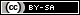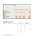# Excel spreadsheet to help with statistics involving pre-decimal UK currency.

## Description

Until 1971 the UK used pounds (£), shillings (s) and pence (d). There were 12 pence in shilling. 20 shillings in a pound 240 pence in a pound. More about decimalisation can be found here http://en.wikipedia.org/wiki/Decimal_Day If basic arithmetic wasn’t enough of a problem, statistics must have been a nightmare. This excel spreadsheet enables the input of up to 300 prices in pounds, shillings and pence and calculates: The Total sum Mean average Standard Deviation Maximum Minimum Upper quartile Lower Quartile Median It also converts all the pounds, shillings and pence data into (today’s) decimal currency. This makes pre-1971 and post-1971 comparisons possible. (Full details of the mathematics behind this are in the Wikipedia article cited above). The excel side of things is a complicated and probably far more complex than it needs to be. Any improvements welcome.

## Toolbox

There are no actions available for this resource.Loading previews...
 MS Excel file Microsoft ExcelDownloadMS Excel file
1 file in this resource